鲤——processing动画交互应用

前言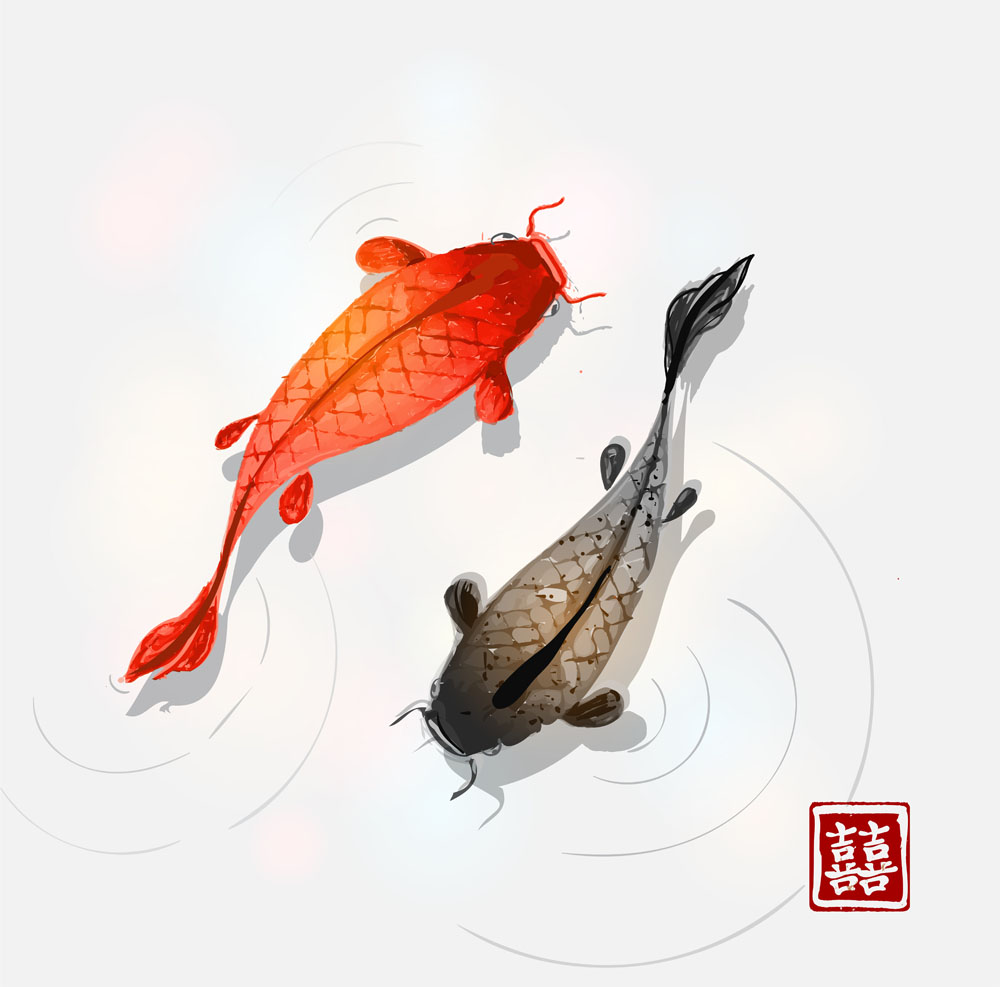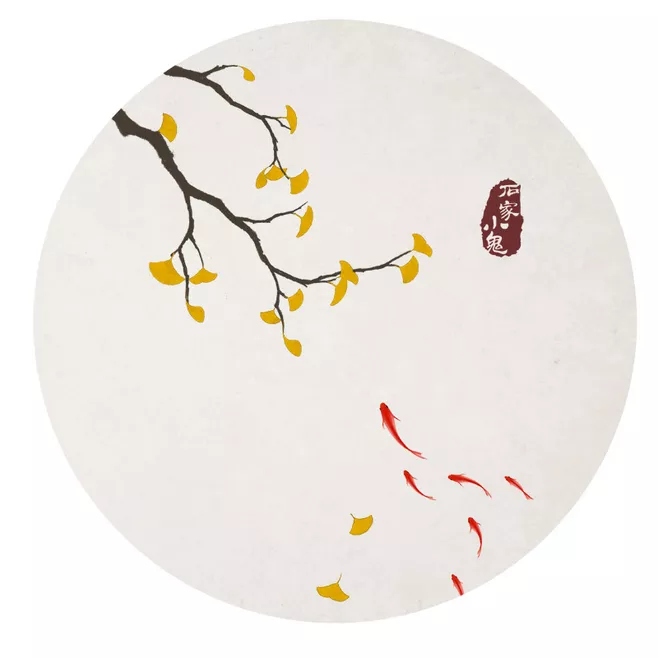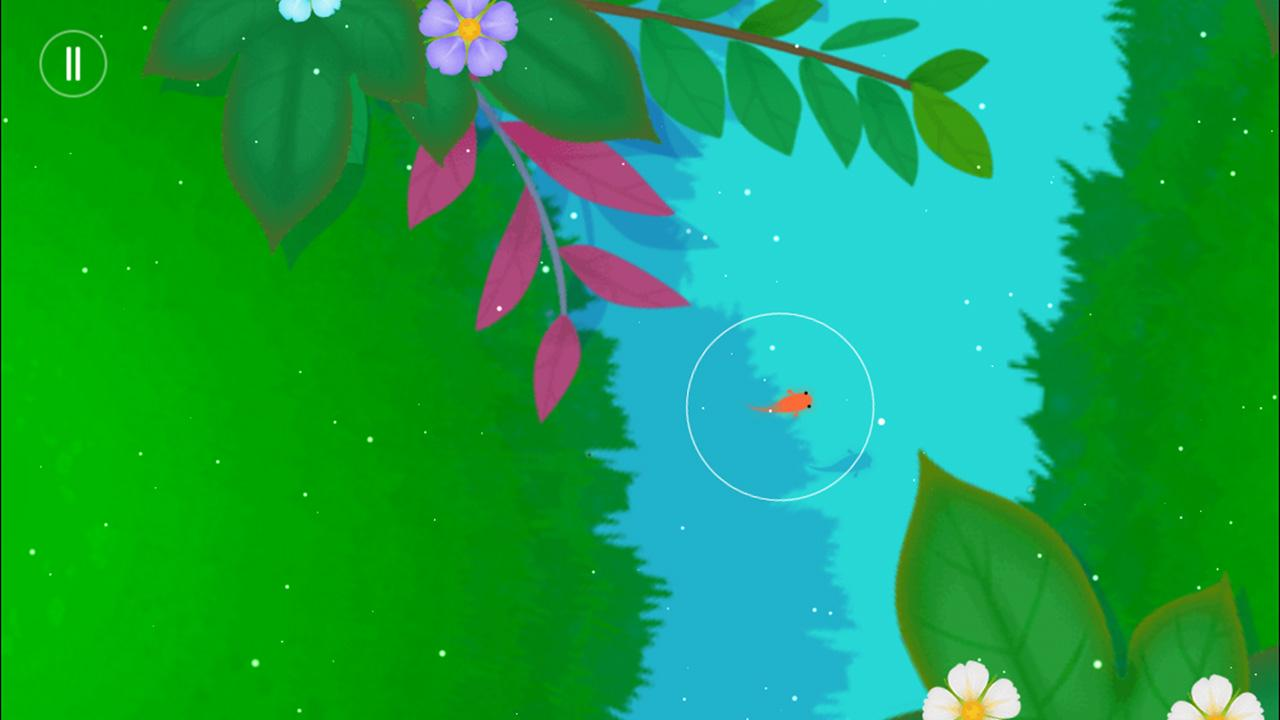应用设计

1.首先是最基本的，鼠标移动控制鲤鱼在池塘中自由游动，并伴有一定的动画效果。
2.鼠标按下时，会给鱼一个当前方向的力，让鱼儿加速。
3.鲤鱼游动时会不断吐出泡泡，泡泡受到浮力与重力影响（浮力肯定是要大一点的）向上移动。
4.随机地在池塘中产生鱼食，产生时会有波纹，鱼食经过一段时间后消失，鲤鱼吃到鱼食时会在当前位置动态出现莲花。
5.鲤鱼最初为白色，每吃一次鱼食就会加深颜色直到最终变红。
6.画面上方有文字提醒，当鲤鱼变成最终心态后赋予一个固定的力让鱼游动，游戏结束。

编写过程

1.虽然我也很想自己设计一个完整的鲤鱼动画…不过时间紧迫加上能力限制，最终使用的鲤鱼蓝本来源于一个开源项目（看到这个项目也促使了我做这个主题），我借鉴了其中绘制鲤鱼的一部分代码，是哪个网站找到的我记不得了…

github源码：https://github.com/dasl-/my-life-aquatic

Boid类(包括我自己的注释):

class Boid {
public PVector location;
public PVector velocity;
private PVector acceleration;
private float maxForce;
private float maxSpeed;

public Boid( PVector _location, float _maxSpeed, float _maxForce) {
createBoid(_location, _maxSpeed, _maxForce);
velocity= new PVector( random( -maxSpeed, maxSpeed ), random( -maxSpeed, maxSpeed ) );
}
private void createBoid(PVector _location, float _maxSpeed, float _maxForce) {
location 		= _location;
maxSpeed 		= _maxSpeed;
maxForce 		= _maxForce;
acceleration 	= new PVector( 0, 0 );
}

protected void update() {
velocity.limit(maxSpeed*2);
acceleration.mult(0);
}

private PVector steer( PVector _target, boolean _slowdown ) {
PVector steer;
PVector desired = PVector.sub( _target, location );//target minus location now
float dist = desired.mag();// get vector length
if ( dist > 0 ) {
desired.normalize();//danweihua vector,make the length=1
if ( _slowdown && dist < 60 ) {
desired.mult( maxSpeed * (dist / 60) );
}
else {
desired.mult( maxSpeed );//direction*speed
}
steer = PVector.sub( desired, velocity );
steer.limit( maxForce );
}
else {
steer =new PVector( 0, 0 );
}
return steer;
}

protected void seek(PVector _target) {
acceleration.add( steer( _target, false ) );
}
}

Boid主要掌管鲤鱼的运动，其中的update和代码本色中的Mover类很相似，由位置，速度，加速度和力的层层累加完成当前时刻鲤鱼位置的计算，不同的是鲤鱼的速度有最大上限，此处最大上限为定义的最大速度（maxspeed）的两倍，原因在下面再解释。这个类被创建时，会定义最初位置，最大速度与最大力并给予一个随机的初始速度。

Fish类继承了Boid类，而Flagellum类主要用于确定每一时刻的鲤鱼各个部分的位置与形状，在Fish类中定义了五个Flagellum类：身体，两半尾巴和两个鱼鳍，Fish类中的render函数负责绘制整个鲤鱼，这些部分我粗略看了之后没怎看懂，后来也没有更多时间 细细看了，有兴趣的朋友可以去下了源码研究研究。Fish的update函数中这一部分承接上文实现鲤鱼的游动：

if(mousePosition.x != mousePositionOld.x ||mousePosition.y != mousePositionOld.y)
seek(mousePosition);//move to the mouse but some bugs...
mousePositionOld = mousePosition;

Fish fish;
PImage p;
public void setup() {
smooth();
size(1400, 900);
fish=new Fish(new PVector(700,50),3,5);
frameRate(45);
}

void drawBackground()
{
image(p,0,0);
}

public void draw() {
drawBackground();
fish.render();
fish.update();

}

public void mouseMoved() {
PVector mousePosition = new PVector(mouseX, mouseY);
fish.setMousePosition(mousePosition);
}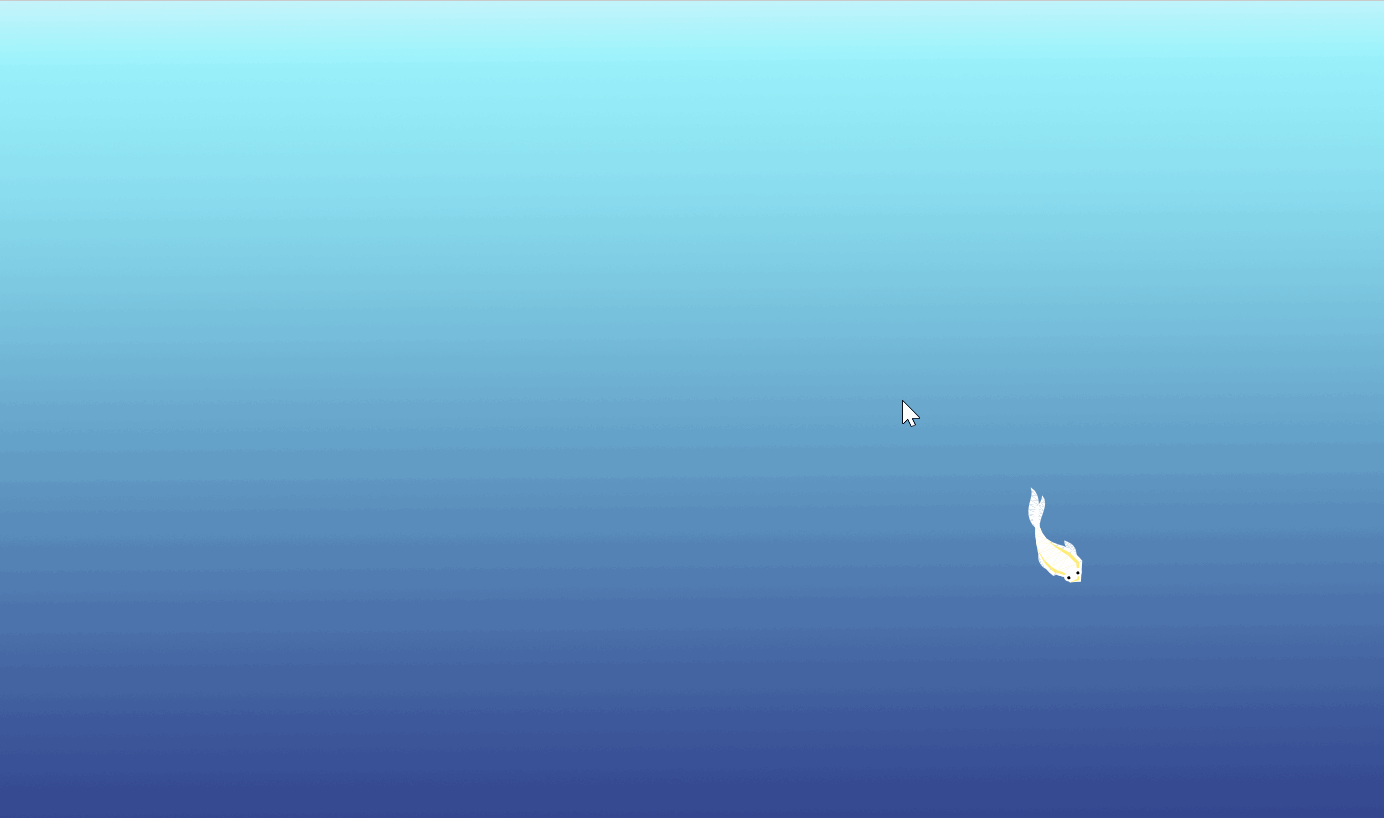2.鼠标点击后施加力做起来还是挺简单的，在Boid里加入两个函数：

public void applyForce() {
PVector f = velocity;
f.normalize();
f.mult(10);
}

public void deleteForce() {
velocity.normalize();
velocity.mult(maxSpeed);
}

if(mousePressed)
{
fish.applyForce();
PVector mousePosition = new PVector(mouseX, mouseY);
fish.setMousePosition(mousePosition);
}
else
{
fish.deleteForce();
}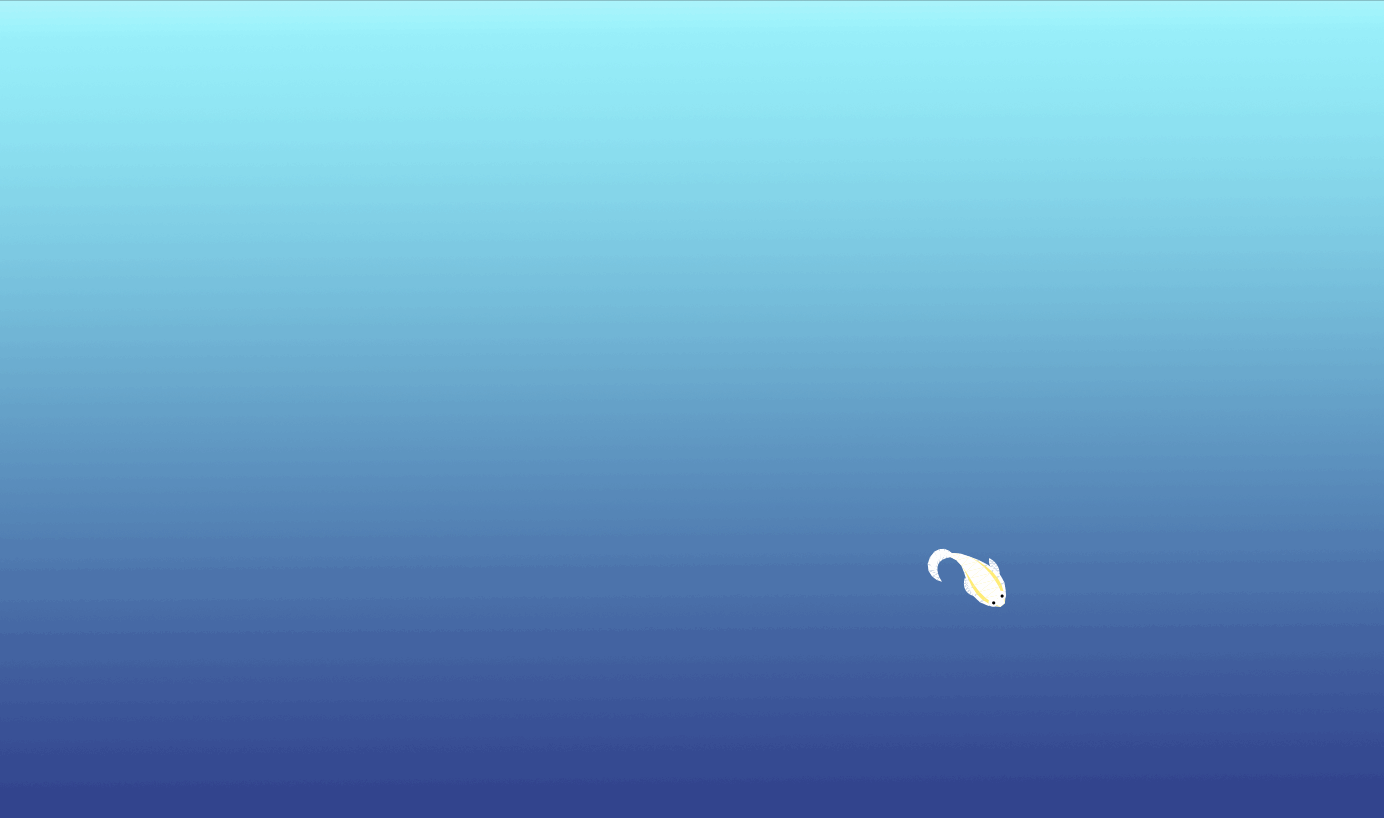3.这一部分要用到粒子系统，基础的粒子类在代码本色里是有的：

class Particle {
PVector position;
PVector velocity;
PVector acceleration;
float lifespan;

Particle(PVector l) {
acceleration = new PVector(0, 0.05);
velocity = new PVector(random(-1, 1), random(-1, 0));
position = new PVector (l.x, l.y);
lifespan = 255.0;
}
void run() {
update();
display();
}
void update() {
acceleration.mult(0);
}
void display() {
}
if (lifespan < 0.0) {
return true;
}
else {
return false;
}
}
}

class Particle1 extends Particle
{
final static float MAX_SPEED = 0.1;
PVector vel = new PVector(random(-MAX_SPEED, MAX_SPEED), random(-MAX_SPEED, MAX_SPEED));
PVector acc = new PVector(0, 0);
PVector pos;
float mass = random(1, 2);
float size = mass*random(3,4);
int lifespan = 255;
color c;
float wei;
Particle1(PVector p)
{
super(p);
pos = new PVector (p.x, p.y);
acc = new PVector (random(0.1, 1.5), 0);
if(random(0,1)<0.5)
c=#ffffff;
else
c=#1E90FF;
wei=random(1,3);
}
public void update()
{
acc.mult(0);
size += 0.05;
lifespan=lifespan-2;
}
public void applyForce(PVector force)
{
PVector f = PVector.div(force, mass);
}
public void display()
{
pushMatrix();
noFill();
strokeWeight(wei);
stroke(c,lifespan);
ellipse(pos.x, pos.y, size * 4, size * 4);
popMatrix();
}

}

import java.util.Iterator;
class ParticleSystem1
{
ArrayList<Particle1> particles = new ArrayList<Particle1>();
void update()
{
Iterator<Particle1> i = particles.iterator();
while (i.hasNext()) {
Particle1 p = i.next();
if (p.pos.x > width || p.pos.x < 0) {
i.remove();
continue;
} else if (p.pos.y > height || p.pos.y < 0) {
i.remove();
continue;
}
p.applyForce(new PVector(random(-1,1),0));
p.applyForce(new PVector(0,(p.mass-p.size/3)/3));
p.update();
i.remove();
} else {
p.display();
}
}
}
}

ParticleSystem1 system = new ParticleSystem1();
system.update();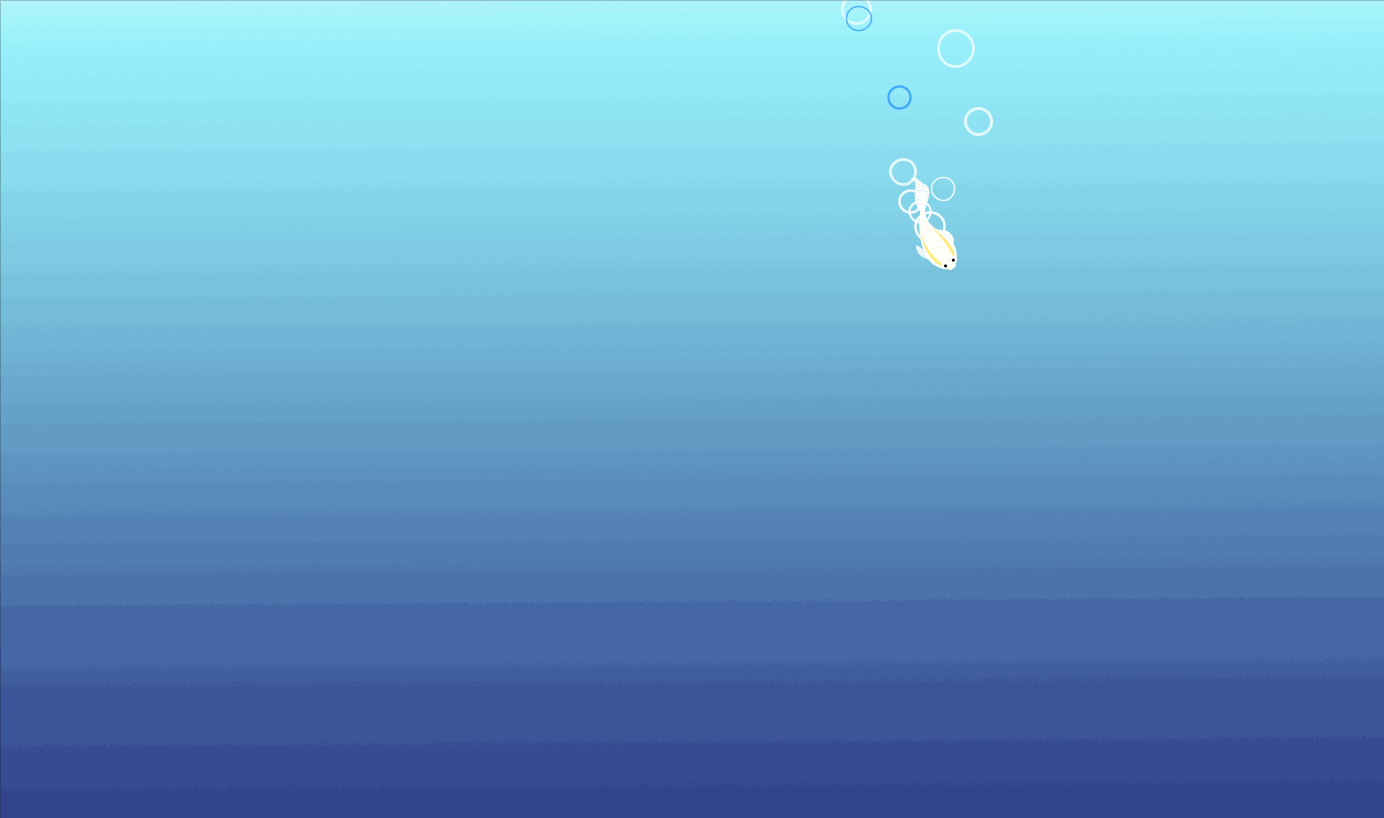4.出现鱼食也是利用粒子系统，基本架构和前面一样我就不解释贴代码了，鱼食是一个个出现的，同一时间只有一个，鱼食出现的地方会有简单的波纹，鱼食在原地一段时间后会移动并缩小。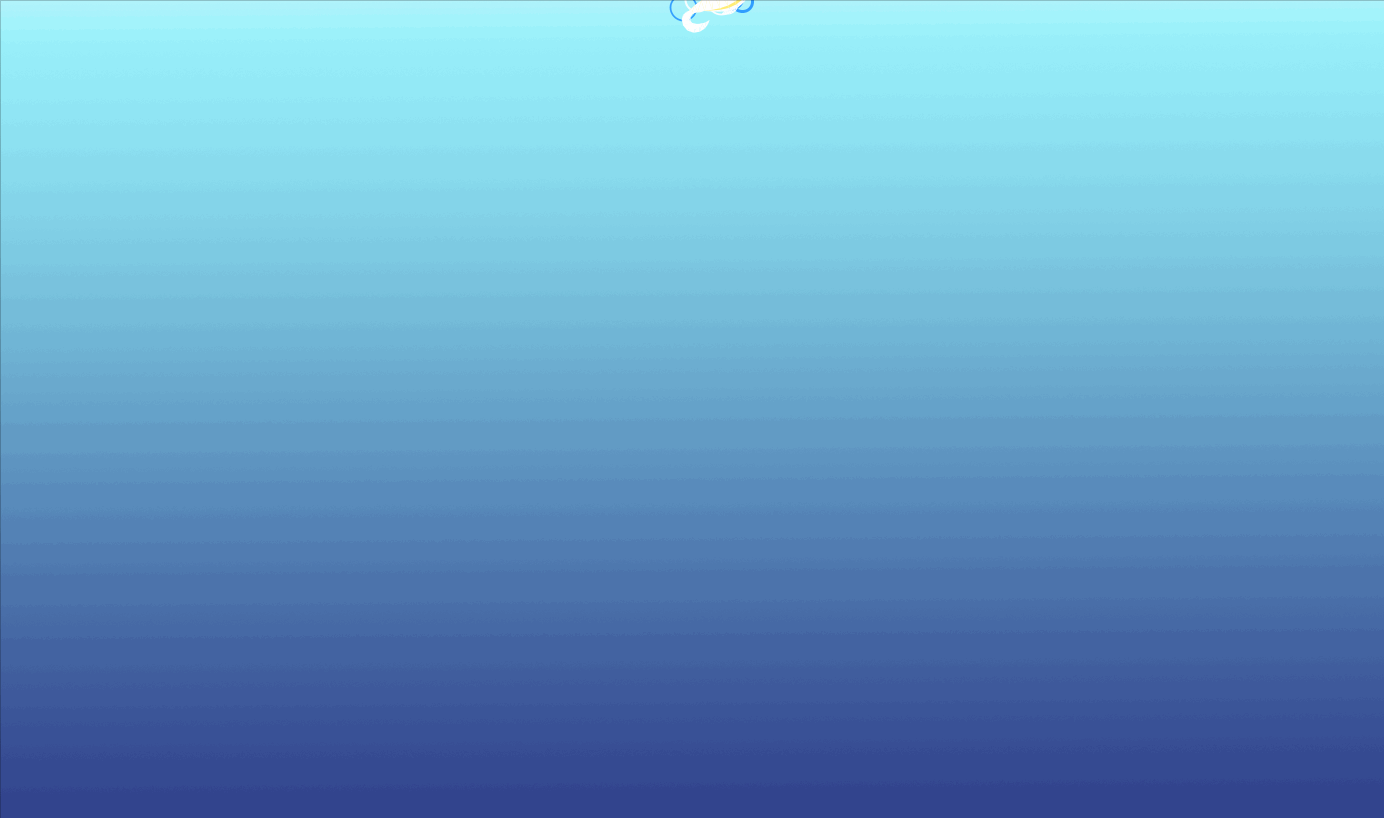pushMatrix();
fill(255);
stroke(255,192,200);
strokeWeight(5);
translate(pos.x, pos.y);
scale(0.5);
ellipse(0,0,120, 120);
rotate(2*angle);
for (int i=0; i<16; i++) {
pushMatrix();
float i0=30;
rotate(i* TWO_PI/16);
translate(0, i0);
ellipse(0, 0, 30, 30);
noFill();
stroke(255,192,200);
beginShape();
vertex(0,0);
bezierVertex(10,50,30,90,100,100);
bezierVertex(90,30,50,10,0,0);
endShape();
popMatrix();
}
if(angle<150*PI)
angle+=speed;
else
;
popMatrix();

if((Math.abs(fish.location.x-foodnow.pos.x)<20)&&(Math.abs(fish.location.y-foodnow.pos.y)<20)&&(foodnow.lifespan>0))
{
foodnow.lifespan=0;
}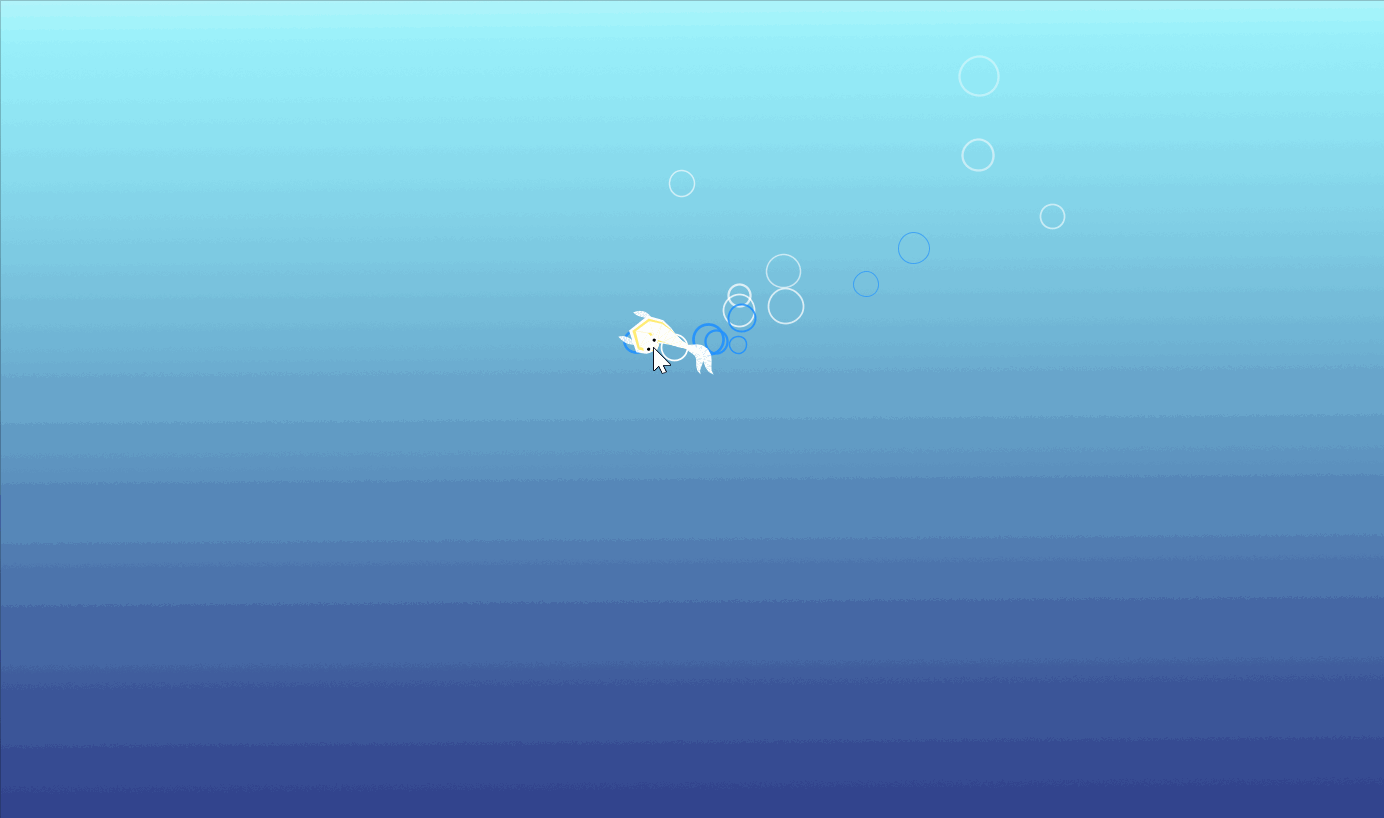5.鱼的颜色加深实现起来很简单，每次判断吃到鱼食时改变鱼的颜色就行。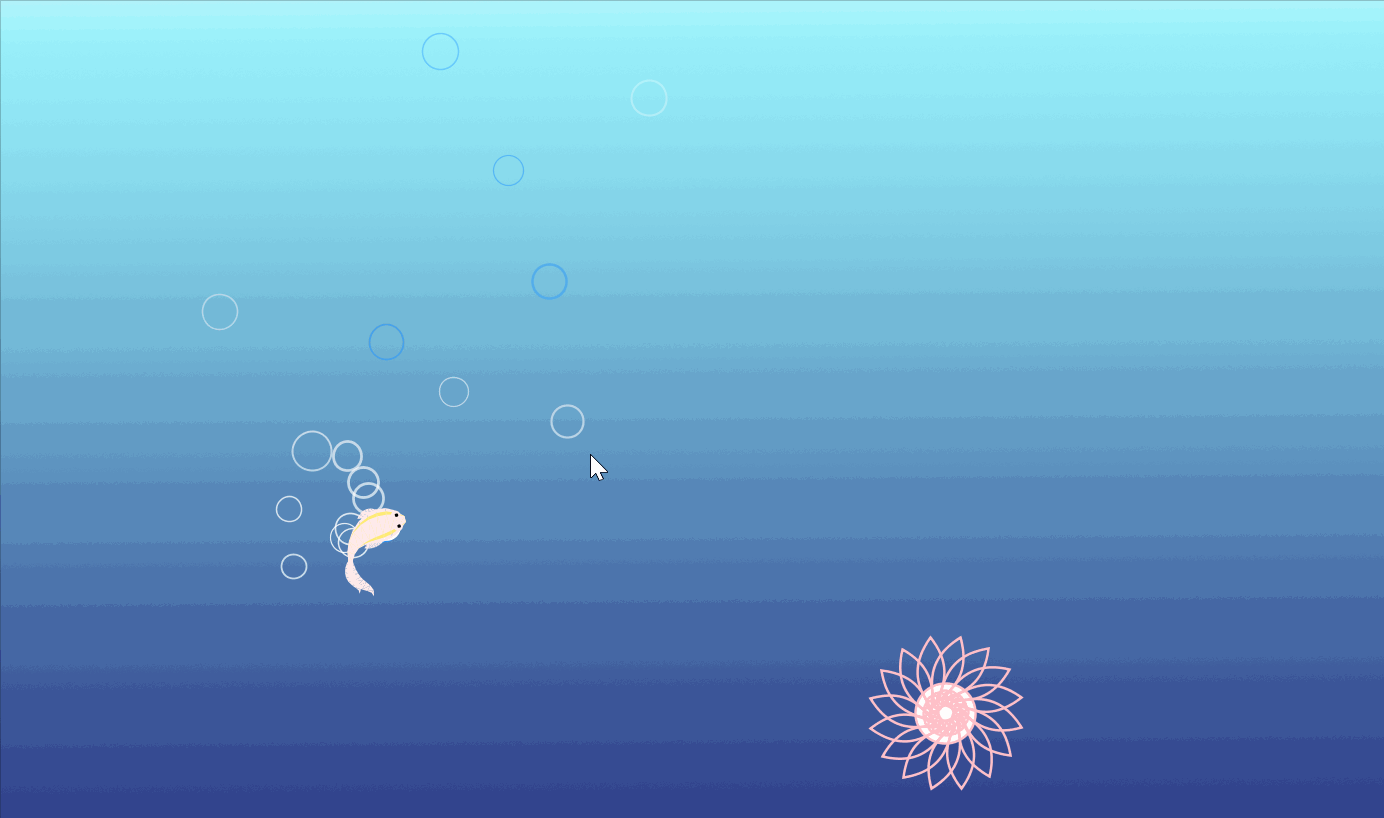6.最后是一个简单的文字提示和特效，鱼儿最终变为红色时会改变最上方的文字，初始文字和最后文字都有一点简单的动画效果。
text类：

class Text {
char text;
float homex, homey;
float x, y;
float theta;
Text(float x1, float y1, char text1) {
homex = x = x1;
homey = y = y1;
text = text1;
x = random(width);
y = random(height);
theta = random(TWO_PI);
}

void display() {
textAlign(LEFT);
pushMatrix();
translate(x, y);
rotate(theta);
text(text, 0, 0);
popMatrix();
}
void home() {
x = lerp(x, homex, 0.04);
y = lerp(y, homey, 0.04);
theta = lerp(theta, 0, 0.04);
}
}参考资料

1.鲤鱼游动：

github源码：https://github.com/dasl-/my-life-aquatic
2.openprocessing：https://www.openprocessing.org/

应用推荐

1.【融入动画技术的交互应用】——PlayWithThePicture
https://blog.csdn.net/qq_41616984/article/details/89737428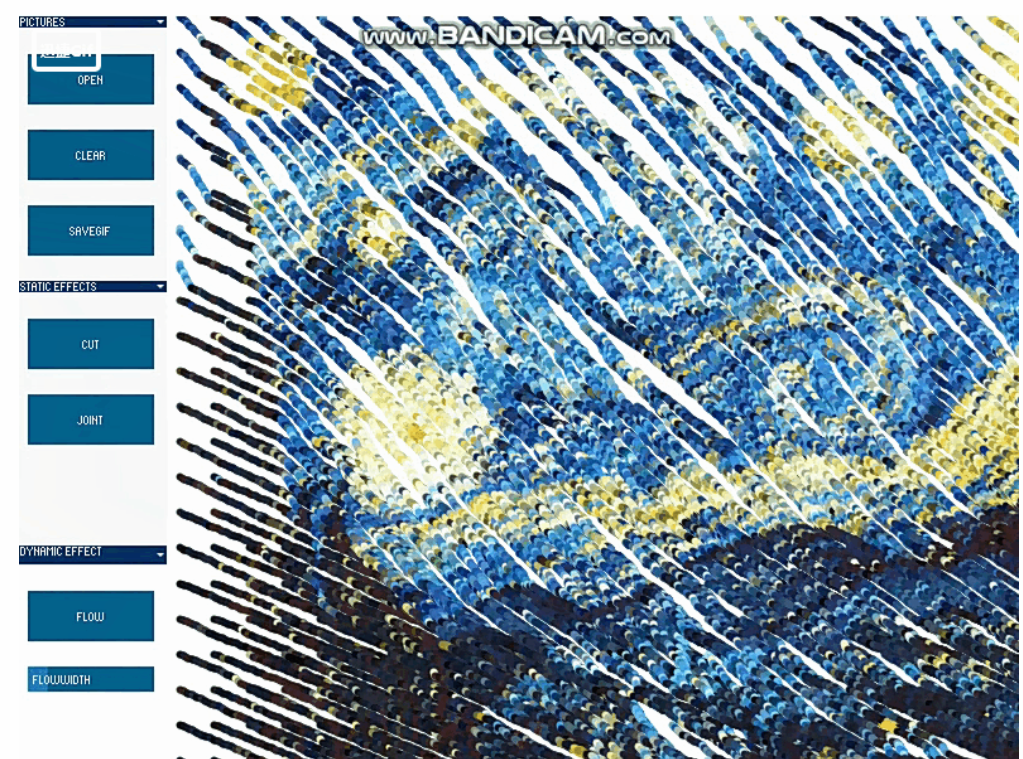2.交互应用 | 音乐坊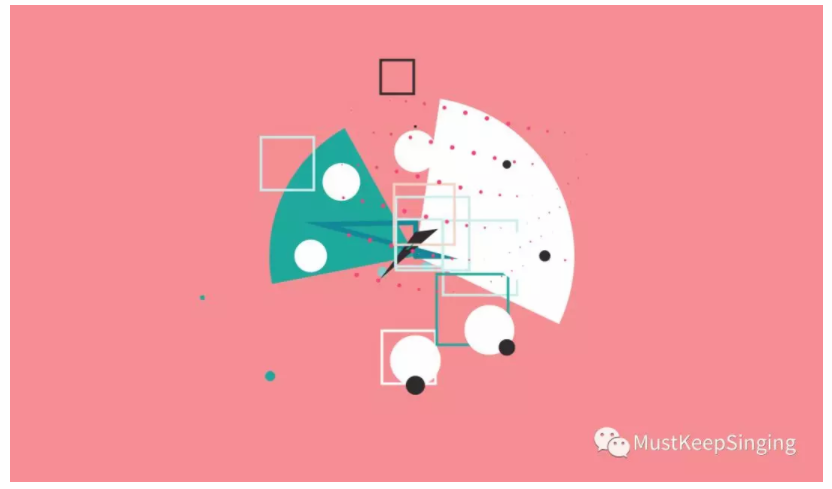3.用Processing制作一个「生态瓶」
https://zhuanlan.zhihu.com/p/64726213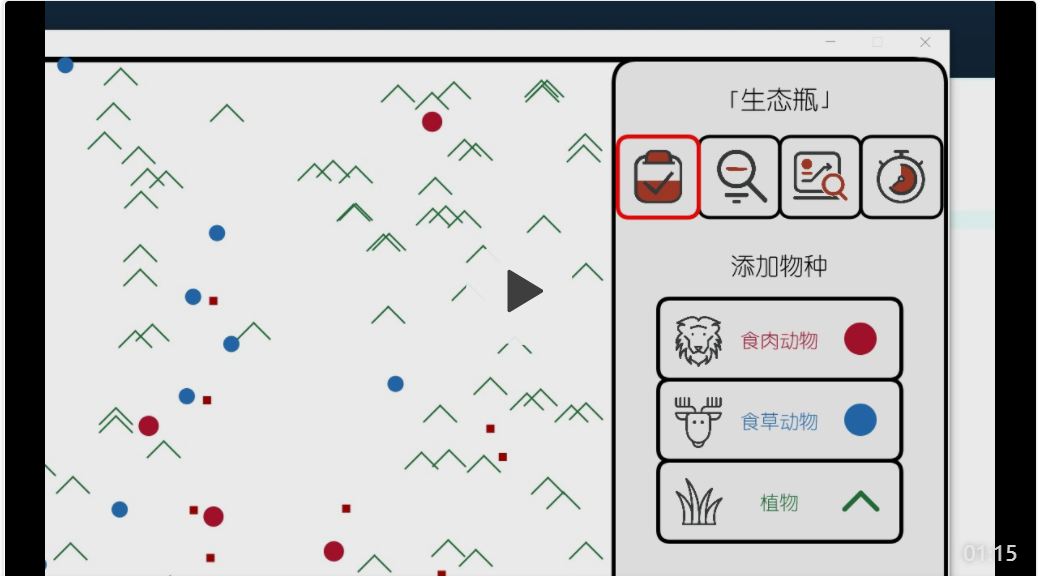10-08
05-0495204-27976
04-259756
11-241万+
11-271万+
11-27536
04-20696
10-212290
05-31
03-111887
12-292868
06-244847
02-26263
11-272955
10-171867
03-26¥2 ¥4 ¥6 ¥10 ¥20余额支付 (余额：-- )扫码支付获取中扫码支付点击重新获取扫码支付1.余额是钱包充值的虚拟货币，按照1:1的比例进行支付金额的抵扣。
2.余额无法直接购买下载，可以购买VIP、C币套餐、付费专栏及课程。余额充值